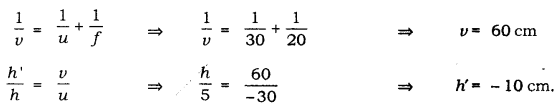# A 5.0cm tall object is placed perpendicular to the principal axis of a convex lens of focal length 20 cm

A 5.0cm tall object is placed perpendicular to the principal axis of a convex lens of focal length 20 cm. The distance of the object from the lens is 30 cm. By calculation determine
(i) the position, and
(ii) the size of the image formed.

We have, Height of the object (h) = 5.0 cm
Focal length (f) = 20 cm 1
Object distance (u) = - 30 cm
Image distance (y) = ?
Height of image (h’) = ?